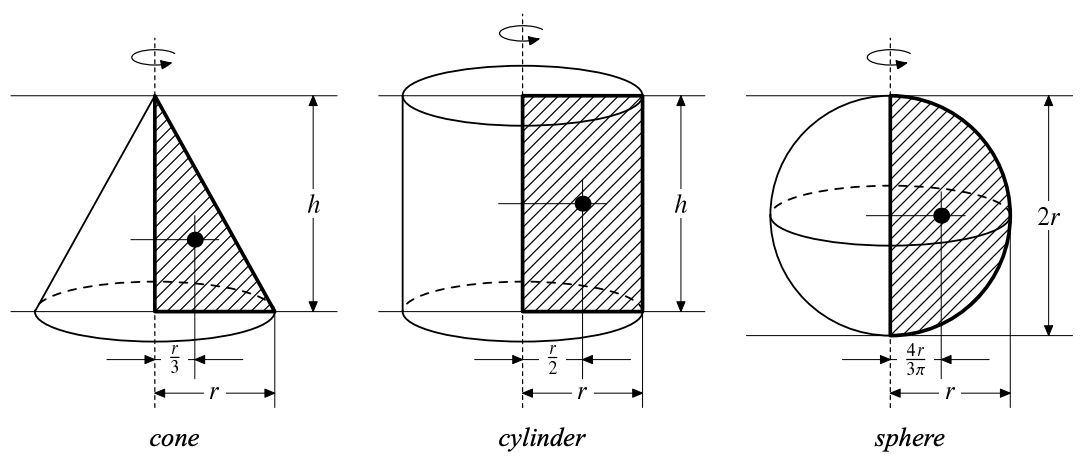시간 제한 메모리 제한 제출 정답 맞은 사람 정답 비율
2 초 512 MB 0 0 0 0.000%

## 문제

Given a convex polygon with N vertices p1, . . . , pN and a line L on a 2-dimensional plane. You can generate a 3-dimensional solid of revolution by the revolution of the convex polygon around the axis L. Now your mission is to calculate the volume of this solid.

When the axis L is an external line of the convex polygon, it’s much easier, because the following theorem will help you. However, you have to note that the axis may intersect the convex polygon.

The second theorem of Pappus: The volume V of a solid of revolution generated by the revolution of a lamina about an external axis is equal to the product of the area A of the lamina and the distance traveled by the lamina’s geometric centroid x.Figure 5: Sample revolutions

## 입력

The first line of the input is a positive integer T, denoting the number of test cases followed. The first line of each test case is a positive integer N (2 < N ≤ 100), which represents the number of vertices of the convex polygon. Then N lines follow. The i-th (1 ≤ i ≤ N) line contains two real numbers Xi (0 ≤ Xi ≤ 10000), Yi (0 ≤ Yi ≤ 10000) which give the coordinates of the vertices of the convex polygon in clockwise order. Finally, there are three real numbers A, B, C (−1000 ≤ A, B,C ≤ 1000) representing the equation of the axis Ax + By + C = 0.

## 출력

The output should consist of T lines, one line for each test case. Each line should contain only one real number which represents the volume V of the solid of revolution, with an error not greater than 0.1.

## 예제 입력 1

2
4
0 0
0 1
1 1
1 0
1 0 0
4
0 0
0 1
1 1
1 0
2 0 -1


## 예제 출력 1

3.1
0.8